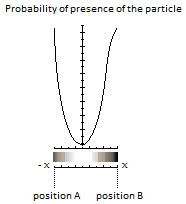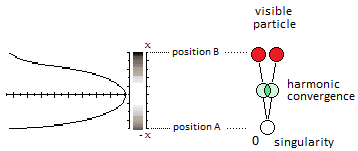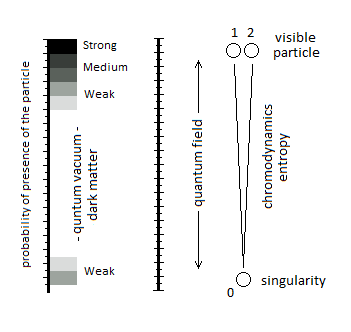# Mass of the Particle and Gluon

## The Quark:

The quark represents one of the two edge of the potential well. It is the maximum point, the end of the length of x axis (position B). At its opposite there is the singularity which represents the other end/edge of the potential well, and which is the maximum point of the length of -x axis (position A). These two positions represent the pure correlated mass.

The mass is given by the near immobility of the particle at its turning point in the potential well.## The Gluon:

During an alternation of two quarks for example, generates in the underlying dynamics a convergence of the two quarks at a single point. Between two is created a harmony of superposition and interaction of the particle which represents the gluon.## The Quantum ChromoDynamics:

The quantum chromodynamics is only a field of interaction that interferes with the particle in its convergence of the relative position of the coupling constant.

For the gluon:
The magnitude of the particle, during the assembly of the quarks, generates the fact that the particle converges in its axes in relation to its point of origin; until you reach the plasma level.

Quantum chromodynamics is represented by the splitting of the particle in space-time, and in relation to the point of origin of the singularity. The chromodynamics belongs to the flow of the particle. Below is the diagram of a three-position system, one of whose positions is the point of origin.____ End ____